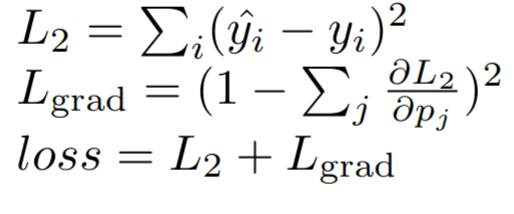# Loss term using gradients

Hi,

I am working on a classification problem using a typical L2 loss. Is there a proper way of adding a loss term which is a function of gradients? For example, lets say I want the gradients of certain layer to sum up to 1:What would be the proper way of implementing that in pytorch?

``````x1 = layer1(inputs)
x2 = layer2(x1)
preds = layer3(x2)

loss_l2 = l2(preds, labels)
loss_l2.backward() # get the gradients